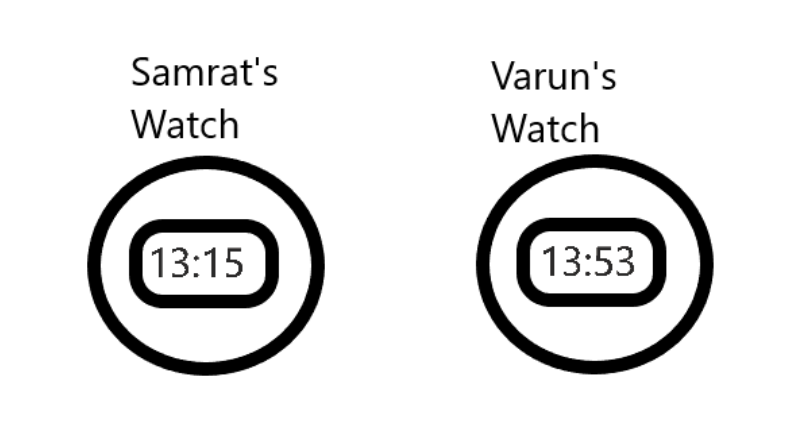Courses
Courses for Kids
Free study material
Free LIVE classes
More# The following time was seen on Samrat and Varun’s watch when the actual time is 1 : 28 p.m. Calculate the time shown on Varun’s watch, when the actual time was half past 6 in the evening.A.5 : 30 p.m.B.6 : 55 p.m.C.6 : 30 p.m.D.5 : 55 p.m.Last updated date: 29th Mar 2023
Total views: 206.7k
Views today: 5.83kVerified
206.7k+ views
Hint: There are different ways we can represent time. In 12 hour format and in 24 hour format.
In a 12 hour format midnight to noon 12 is designated as a.m. and post that 1 to 12 midnight in day is designated as p.m.
In 24 hour format $00{\text{ }}:{\text{ }}00$ is midnight.
$23{\text{ }}:{\text{ }}00$is the 24th hour of the day.

In the given problem Samrat’s watch shows $13{\text{ }}:{\text{ }}15$.
Varun’s watch shows $13{\text{ }}:{\text{ }}53$ both in 24 hour format.
If we convert it into 12 hour format the time in the watches will be $1{\text{ }}:{\text{ }}15{\text{ }}p.m.$ in Samrat’s watch. $1{\text{ }}:{\text{ }}53{\text{ }}p.m.$ in Varun’s watch.
In the question it is given that actual time in the clock in $1{\text{ }}:{\text{ }}28{\text{ }}p.m.$
So, the watches of Samrat and Varun are showing the wrong time. The difference between actual and watch timings
$Samrat's\,watch\,:\,(1:15) - (1:28) \\ \Rightarrow 13\,\min utes\,lagging \\ Varun's\,watch\,:\,(1:53) - (1:28) \\ \Rightarrow 25\,\min utes\,ahead \;$
As per the requirement of question we need to find out what is the time in Varun’s watch when it is actually half past 6 i.e. $6:30\,p.m.$
As we know from the above calculations that the Varun’s watch is 25 minutes ahead
So, the time in Varun’s watch is
$= (6:30) + 25\min utes \\ = 6:55\,p.m. \;$
Hence option (B) is the answer.
So, the correct answer is “Option B”.

Note: The students must understand the difference between 12 hour format and 24 hour format. Accordingly, how the question is used and what is to be given as answer. Reading the question carefully and proceeding for the solution will help.
Conversion between time formats need to be understood and done carefully.